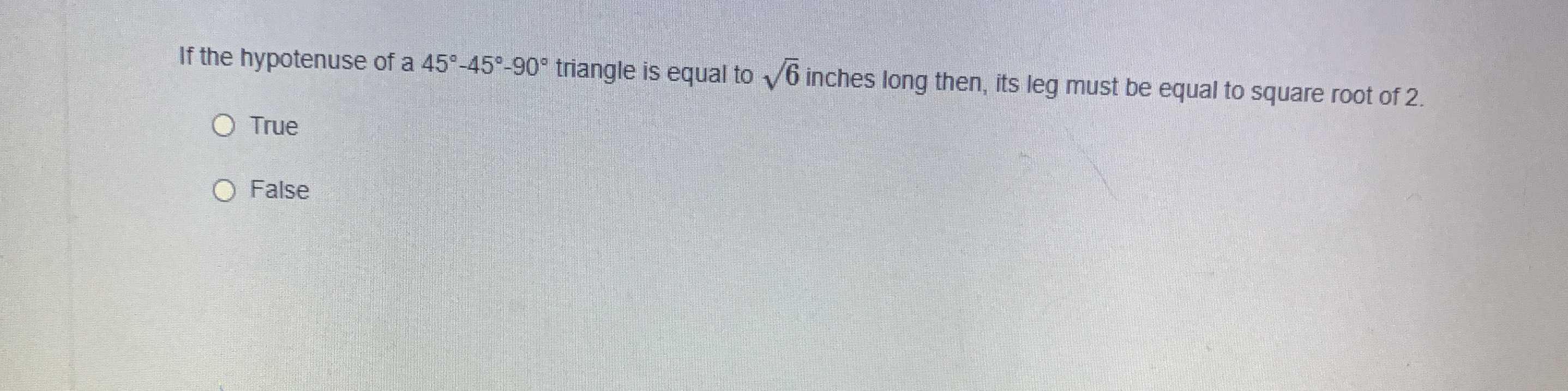### Still have math questions?

Trigonometry
QuestionTrue or false

If the hypotenuse of a $$45 ^ { \circ } - 45 ^ { \circ } - 90 ^ { \circ }$$ triangle is equal to $$\sqrt { 6 }$$ inches long then, its leg must be equal to square root of $$2$$ .

True

False